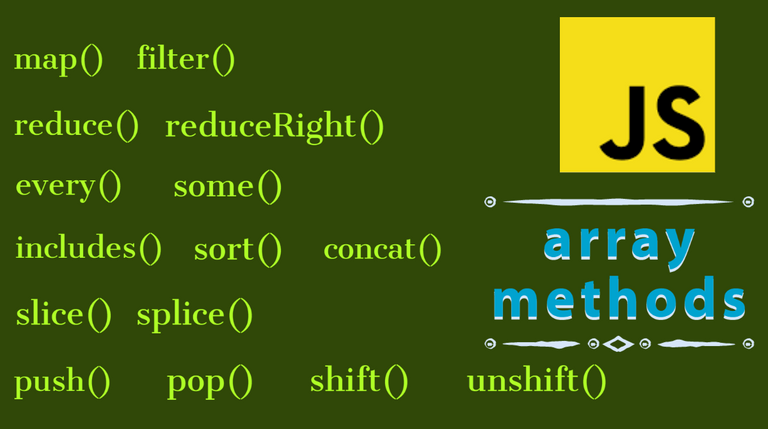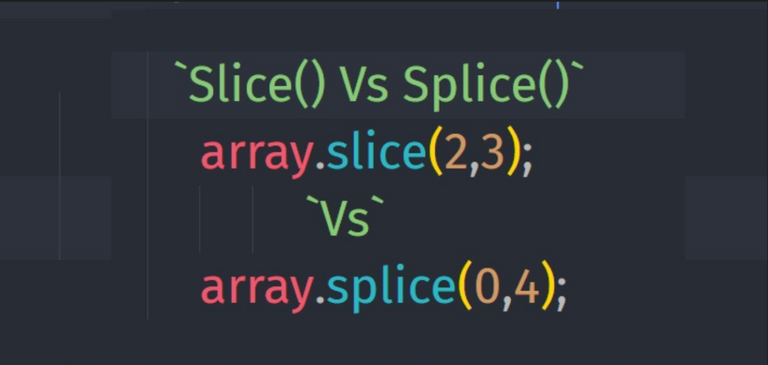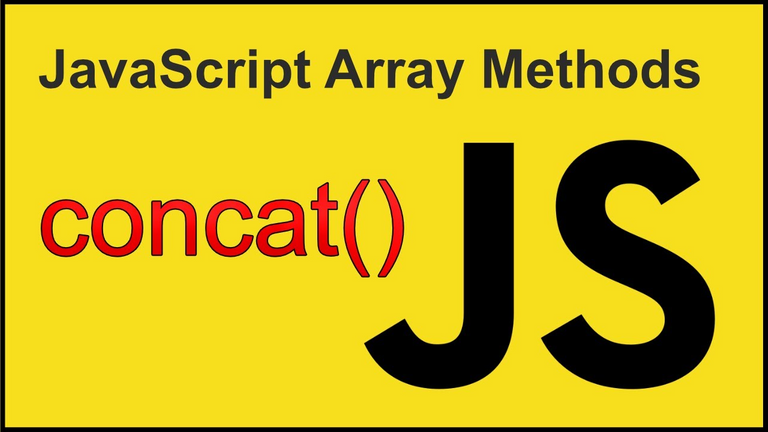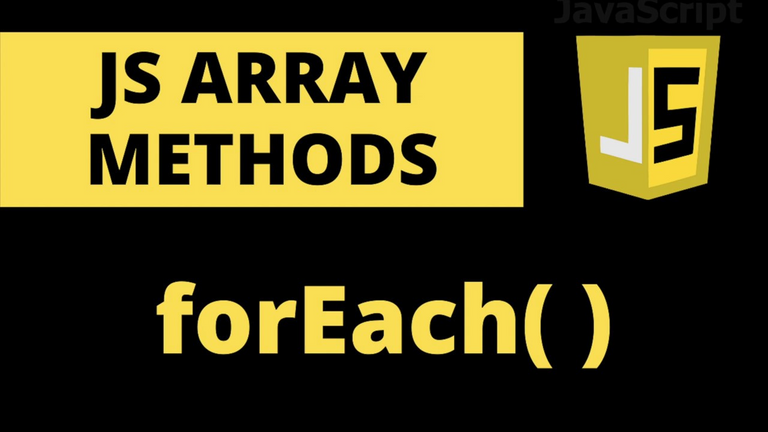# The Full guide to Arrays on Javascript | (1 / x)

in Programming & Dev2 years ago (edited)Arrays are probably the most used Data Structure on Javascript, but it's not only necessary to learn the countless methods they have, we also have to learn how to identify which and when to use each of them. We are here to tackle that topic, brace yourself because there's a huge post incoming, perhaps even three if my calculations are correct.

Why three and not one? My last post regarding JavaScript went through everything there is to know about Functions on JavaScript, but it ended up being way too long for a human being to read it all in one sitting, so I'm going to experiment with the idea of dividing a huge topic like this one intro several chapters, just as I did with the Data Structures topic (which conveniently, talked about arrays, but in a more shallow way, this is the real deal).

# Simple array methodsMethods are simple functions that we can call on objects. They are functions attached to objects, and Arrays are objects. Array methods are built-in into JS, and they are attached to all arrays we create on JavaScript.

## Slice methodWe can extract part of any array without changing the original one.

Arrays have indexes, so we can play with that and use it as a parameter. Inside the parentheses the first parameter is the begin.

``````let arr = ["a", "b", "c", "d", "e"];
console.log(arr.slice(2));
``````

// Output: [ 'c', 'd', 'e' ]

This doesn't mutate the original array, it returns a new array but only with the extracted parts begining at the index que pass it.

Check out what happens when we pass a begin and end parameter. Remember the end parameter is not included in the output:

``````console.log(arr.slice(2, 4));
``````

Output: [ 'c', 'd' ]

We can also pass into the method negative parameters so we start counting from the end:

``````console.log(arr.slice(-2));
``````

Output: [ 'd', 'e' ]

When we pass a negative parameter as the end parameter, the slice method doesn't take that index for the new array, it stops there but doesn't include it.

``````console.log(arr.slice(1, -2));
``````

Output: [ 'b', 'c' ]

We can use the .slice method to create a shallow copy of any array, all we need to do is pass the slice method with no arguments. This is very similar to the spread operator, they work pretty much the same, the only difference is that this method can be chained with other methods, and the spread operator can't.

``````console.log(arr.slice());
``````

Output: [ 'a', 'b', 'c', 'd', 'e' ]

``````console.log([...arr]);
``````

Output: [ 'a', 'b', 'c', 'd', 'e' ]

## Splice method

This method works exactly as the slice method, but it mutates the original array, and returns the original array with the changes you passed in.

The splice method is basically a way to delete or remove elements from an array.

``````console.log(arr.splice(2)); // Output: [ 'c', 'd', 'e' ]
console.log(arr); // Output: [ 'a', 'b' ]
``````

As you can see, the splice method returned an array with the elements starting at the index 2 til the end, but it mutated the original array which is now composed only by the first and second index elements. The splice method deletes the extracted elements.

We can delete as many elements from an array, we just need to use the begin and end parameters correctly.

We have two parameters in the array.splice method, the first one is the begin parameter, which is where the splice will begin; the second one is the delete count which passes the number of elements we want to remove from the array.

``````let array = ["a", "b", "c", "d", "e"];
array.splice(1, 3);
console.log(array);
``````

Output: [ 'a', 'e' ]

## Reverse ArraysReversing an array using the method .reverse is quite simple, all we have to remember is that the original array is mutated, no new array is created through this method.

``````const hiveUSers = ["h", "i", "j", "k", "l", "m"];
hiveUSers.reverse();
console.log(hiveUSers);
``````

Output: [ 'm', 'l', 'k', 'j', 'i', 'h' ]

## Concatenating two arraysAs the name says, this method allows us to concatenate two arrays into a new array that is created when we call the method.

``````const abc = ["a", "b", "c"];
const def = ["d", "e", "f"];
const abcdef = abc.concat(def);
console.log(abcdef);
``````

Output: [ 'a', 'b', 'c', 'd', 'e', 'f' ]

As you can see, this array method is pretty similar to when using the spread operator like this:

``````console.log([...abc, ...def]);
``````

Ouput: [ 'a', 'b', 'c', 'd', 'e', 'f' ]

Both ways work the same, but as I mentioned before, the spread operator cannot be used with other method whereas the .concat method can be chained.

## Joining two arrays

This one speaks for itself, and it is kind of silly to exemplify it but just for the sake of it, let's do it.

Something to note in the output: The result is no longer an array but a string.

``````console.log(abcdef.join());
``````

Output: a,b,c,d,e,f

The strings are divided by a comma, but we can actually pass anything we want so that the divider is whatever we want it to be:

``````console.log(abcdef.join('-'));
``````

Output: a-b-c-d-e-f

## Looping Arrays: forEachIn previous posts I already talked about the for loop and the forOf loop, this one is new, but the logic stays the same, but has significant differences.

This will be fun, I will create an Exchange simulator while teaching you how to play with arrays.

For every transaction that is made, we will print a message. The positive values are deposits, the negative are withdraws.

``````const hiveTransactions = [100, 50, -90];
for (const transaction of hiveTransactions) {
if (transaction > 0) {
console.log(`You deposited \${transaction} hive`);
} else {
console.log(`You withdrew \${Math.abs(transaction)} hive`); // 1
}
}
``````

1.We use the absolute math function to remove the minus sign.
Output:
You deposited 100 hive
You deposited 50 hive
You withdrew 90 hive*/

Using the forEach method makes the process easier, but that's up to you.

forEach is technically a higher order function, which means that it needs another function (a callback function) to tell it what to do.

Below, it is the forEach method that will call the function right next to the method, not ourselves.

As the forEach method calls the callback function in each iteration, it will pass in the current element of the array as an argument (which is why I specified (transaction), so it's clearer what I mean)

``````hiveTransactions.forEach(function (transaction) {   // 1
if (transaction > 0) {
console.log(`You deposited \${transaction} hive`);
} else {
console.log(`You withdrew \${Math.abs(transaction)} hive`);
}
});
``````

1.We can write anything or leave it empty instead of the parentheses
Output:
You deposited 100 hive
You deposited 50 hive
You withdrew 90 hive

In the function above, we tell the forEach method that in each iteration of the loop it should log one of the two strings we set in the case statement. So in short, we give the forEach method instructions by giving it a callback function, which contains these instructions.

Using the forEach method to solve this issue is important because this logic will help us solve more complicated problems.

Let's say we actually need access to the counter variable for the loop? Just like we can access each index in the forOf loop method, we can access the counter variable in the forEach loop method.

``````const hiveTransactions = [100, 50, -90];
for (const [i, transaction] of hiveTransactions.entries()) {   // 1
if (transaction > 0) {
console.log(`Transaction \${i + 1}: You deposited \${transaction} hive`);
} else {
console.log(
`Transaction \${i + 1}: You withdrew \${Math.abs(transaction)} hive`
);
}
}
``````

1.This is how we access the counter variable in the forOf loop method, specifying the name of the counter for the index looped in each iteration.
Output:
Transaction 1: You deposited 100 hive
Transaction 2: You deposited 50 hive
Transaction 3: You withdrew 90 hive

We can also access the counter variable using the forEach loop method, we'll do it in a second, first some concepts and theory.

It is the forEach method that calls the function inside the parentheses on each iteration and as it call this function, it also passes in the current element of the array, but that is not the only thing that passes, it also passes the index and the whole array, we just need to know how and what to tell the method to pass in besides the current element through which is looping.

We can use one, two or the three arguments. The keyword doesn't matter, what matters is the order. The first parameter is always the current element, the second is the current index, and the third one is the entire array that we are looping order. That is the pre-established order JS works with.

``````hiveTransactions.forEach(function (transaction, index, array) {
// We can write anything or leave it empty instead of the parentheses
if (transaction > 0) {
console.log(`Transaction \${index + 1}: You deposited \${transaction} hive`);
} else {
console.log(
`Transaction \${index + 1}: You withdrew \${Math.abs(transaction)} hive`
);
}
});
``````

Output:
Transaction 1: You deposited 100 hive
Transaction 2: You deposited 50 hive
Transaction 3: You withdrew 90 hive

Notice that in the forOf loop, the first parameter is the index and then the transaction, whereas in the forEach method, the first parameter we pass is the transaction and the second is the index.

It is pretty easy to identify when to use forOf and forEach. In the forEach loops you can't break the loop, it will always run through the entire array, this means the continue and break statements don't work with this loop. If you want to be able to break the loop, you have to use the forOf method.

## Using the forEach method with Maps and Sets

This method is available for maps and sets. Take the next map for example. Remember that maps take a key and value, divided by a comma.

``````const tokens = new Map([
["BTC", "Bitcoin"],
["LTC", "Litecoin"],
["HBD", "Hive Backed Dollar"],
]);

tokens.forEach(function (value, key, map) {   // 1
console.log(`\${key}:\${value}`); //   2
});
``````

1.In arrays we have the current element on the loop, then the index, then the whole array, remember?
2.This is just to provide proof that the elements above indeed exist.
Output:
BTC:Bitcoin
LTC:Litecoin
HBD:Hive Backed Dollar

Remember that sets are similar to maps but they only hold unique values.
In the case of sets, we have to pass an iterable to the set inside the parentheses right after the word set.

``````const tokensUnique = new Set(["BTC", "LTC", "HBD"]);
tokensUnique.forEach(function (value, key, map) {
// The callback function has the same parameters as a map
console.log(`\${key}:\${value}`);
});
``````

Output:
BTC:BTC
LTC:LTC
HBD:HBD

The key is the same as the value. A set doesn't have keys or indexes, so there actually are no values, but if we pass the second argument it still works. This is designed like this (to have the same signature for maps and sets and have three arguments) so it is easier for devs to avoid confusion or errors.

There you go, we are beginning to talk more advanced stuff regarding arrays, I would say we are still at the start of the intermediate part, but hopefully after this series of Array-related posts we (that is me and you, lone reader who still reads my coding posts) will be around advanced level regarding Arrays on JavaScript.

Pictures are not mine, I'm not a designer and can't create images so kickass like those above, here's the source for each of them. In fact, if you follow the links you'll find useful tutorials about that specific topic!

Arrays
Array methods
Slice vs Splice
Reverse method
Concatenate
forEach loopmethod